# Finding Data with Range Names in Same Path

This page explains how you can look in a directory for data that has the same range names.  Say for example that you have a whole lot of models in a single directory and each file has the same range names. For example, each file may have a range name with min_DSCR or equity_IRR.  Alternatively, each file may have array range names with data for a bunch of years.  Let’s say that you want to collect data from each file and accumulate the data in a single file.  This page shows you a macro to accomplish this.  Unlike other situations where I download a single file, in this case you can download an entire zipped directory. When you download the zipped file attached to the button below, put the zipped file in a directory and unzip the file.  Then you open the file, Find Range Names2.xlsm.  This file contains the macros that accumulate the data.

## Introduction and List of Files

When you open the file, you should see something like this.  You can first test if the directory contains the file that you are testing and that directory name is correct.  You can press the button shown in the screenshot below named “Get List of Files”.  In this example, the file itself is in the folder you can use the FIND and the MID functions to make the name of the directory.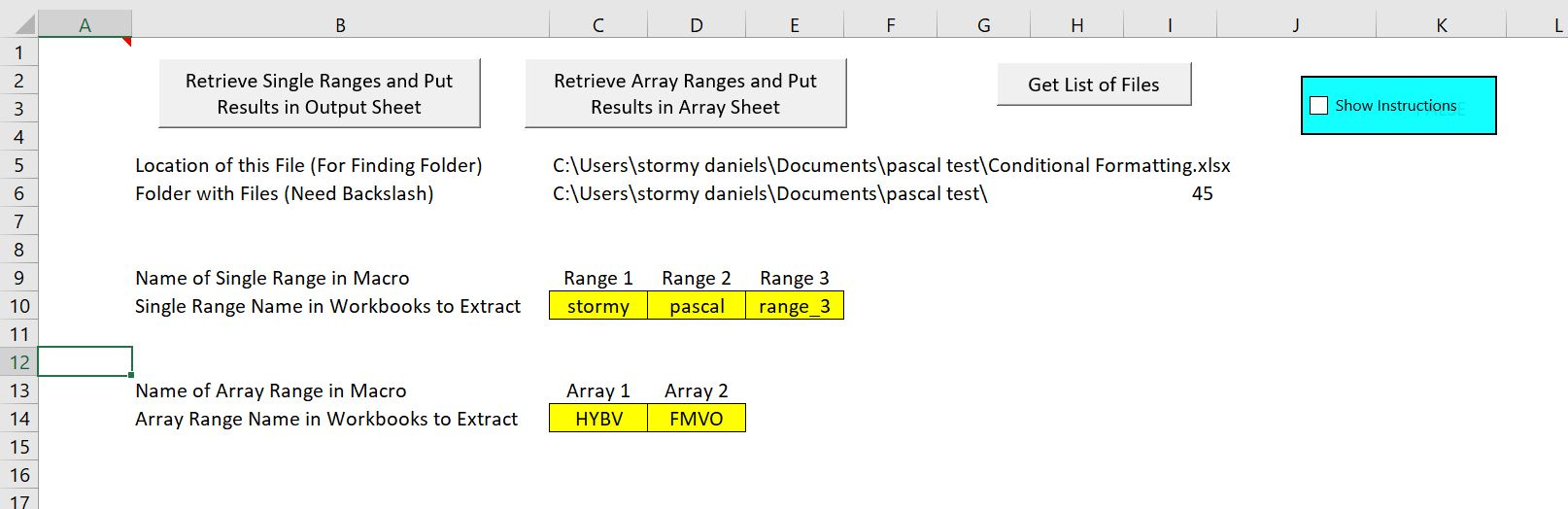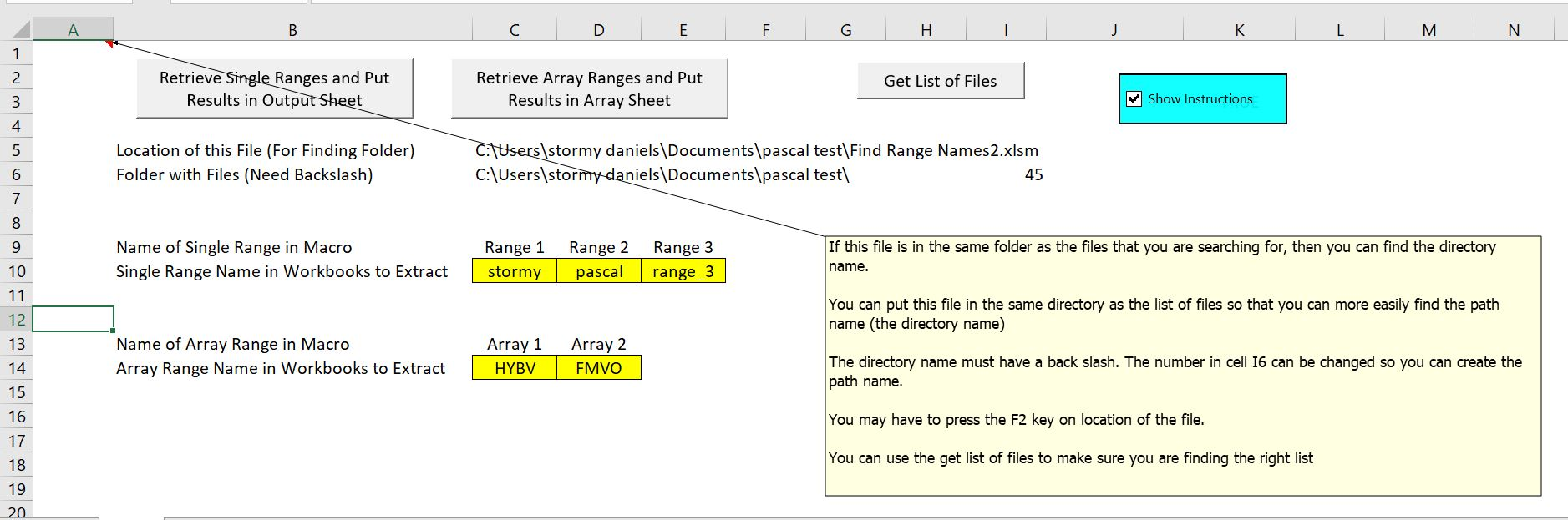The macro to list the files is listed below.  The big deal about this is that you make a loop around files in the directory.

.

```Sub Get_list_of_files()

Application.ScreenUpdating = False

Dim filename As String

base_book = ActiveWorkbook.Name ' name of this book so you can go back

directory_name = Range("directory_name") ' get the directory name from the spreadsheet

MsgBox " This puts the files that are in " & directory_name

' loop through each file name and open it if the extension is correct

filename = Dir(directory_name & "*.xl*") ' this is a big deal --> look in the directory for excel files

Count = 1 ' Count the number of files for the output

While filename <> ""

workbookname = filename

Workbooks(base_book).Sheets("File List").Cells(Count + 2, 2) = Count
Workbooks(base_book).Sheets("File List").Cells(Count + 2, 3) = workbookname

Count = Count + 1

filename = Dir() ' This is also a big deal -- get the next file name

Wend

End Sub```

.## Extracting Data in Single Range Names

The next section shows how to get data when the data is defined by a single range name.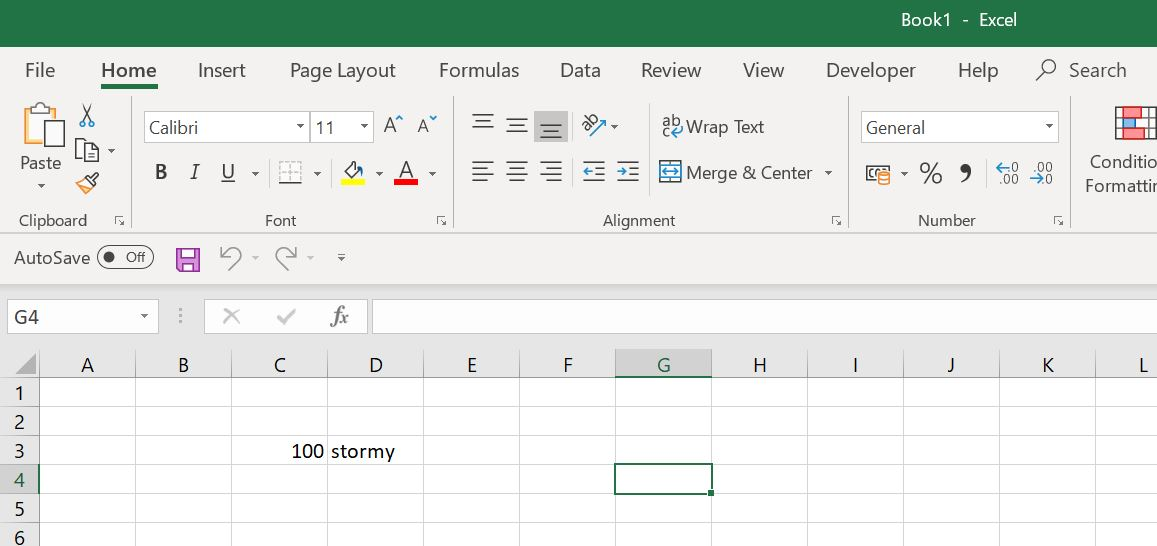.

```Sub Find_Single_range()

Application.DisplayStatusBar = True
Application.EnableEvents = True

Dim sFile As String 'File Name
Dim filename, directory_name As String
Dim range1, range2, range3 As String
Dim result1, result2, result3 As Variant

debug_switch = Range("debug_switch")

Application.ScreenUpdating = True ' choose to not show what is going on
If debug_switch = False Then Application.ScreenUpdating = False ' shows what is happening when macro is running

base_book = ActiveWorkbook.Name ' name of this book so you can go back to the file and print etc.
base_sheet = ActiveSheet.Name ' name of this sheet so you can go back to the file and print etc.

directory_name = Range("directory_name")

' loop through each file name and open it if the extension is correct

Count = 0

range1 = Range("range1")
range2 = Range("range2")
range3 = Range("range3")

' This clears the output range (you must be in the sheet to remove this

Sheets("Output").Select
Range(Cells(Count + Range("start_row"), Range("start_col")), Cells(Count + Range("start_row") + 20, Range("start_col") + 20)).ClearContents
Sheets(base_sheet).Select

filename = Dir(directory_name & "*.xl*") ' Look of all excel files

' arg = "'" & directory_name & "[" & filename & "]" & Sheet & "'!" & Range(ref).Range("A1").Address(, , xlR1C1)

While filename <> "" ' loops around each file in the folder that is defined; when there is no more, the loop is finished

' This defines the name that is used in EeecuteExcel4Macro to get the data

mydata1 = "'" & directory_name & filename & "'!" & range1
mydata2 = "'" & directory_name & filename & "'!" & range2
mydata3 = "'" & directory_name & filename & "'!" & range3

DoEvents

Application.StatusBar = " Range Name 1 " & mydata1 & " In file -- " & filename

' this is the thing that retrieves the data

result1 = ExecuteExcel4Macro(mydata1)
result2 = Application.ExecuteExcel4Macro(mydata2)
result3 = Application.ExecuteExcel4Macro(mydata3)

On Error Resume Next
If result1 = "#NAME?" Then result1 = ""
If result2 = "#NAME?" Then result2 = ""
If result3 = "#NAME?" Then result3 = ""

' MsgBox " Result 1 " & mydata1 & " " & range1 & " " & result1
' MsgBox " Result 2 " & mydata2 & " " & range2 & " " & result2
' MsgBox " Result 3 " & mydata3 & " " & range3 & " " & result3
' result1 = GetValue(directory_name, filename, range1)

workbookname = filename

range1_output = result1
range2_output = result2
range3_output = result3

Workbooks(base_book).Activate ' now go back to the base file to get the next item

If workbookname <> base_book Then

Sheets("Output").Select

Workbooks(base_book).Sheets("Output").Cells(2, Range("start_col") + 1) = directory_name

Workbooks(base_book).Sheets("Output").Cells(Count + Range("start_row"), Range("start_col") + 1) = workbookname

Workbooks(base_book).Sheets("Output").Cells(Count + Range("start_row"), Range("start_col") + 2) = range1_output
Workbooks(base_book).Sheets("Output").Cells(Count + Range("start_row"), Range("start_col") + 3) = range2_output
Workbooks(base_book).Sheets("Output").Cells(Count + Range("start_row"), Range("start_col") + 4) = range3_output

End If

filename = Dir() ' Define the next file name in the directory

Count = Count + 1 ' Count for the output

Wend

after_while:

Workbooks(base_book).Activate

Application.StatusBar = False

End Sub```

.

## Reading Data Defined by Array Range Name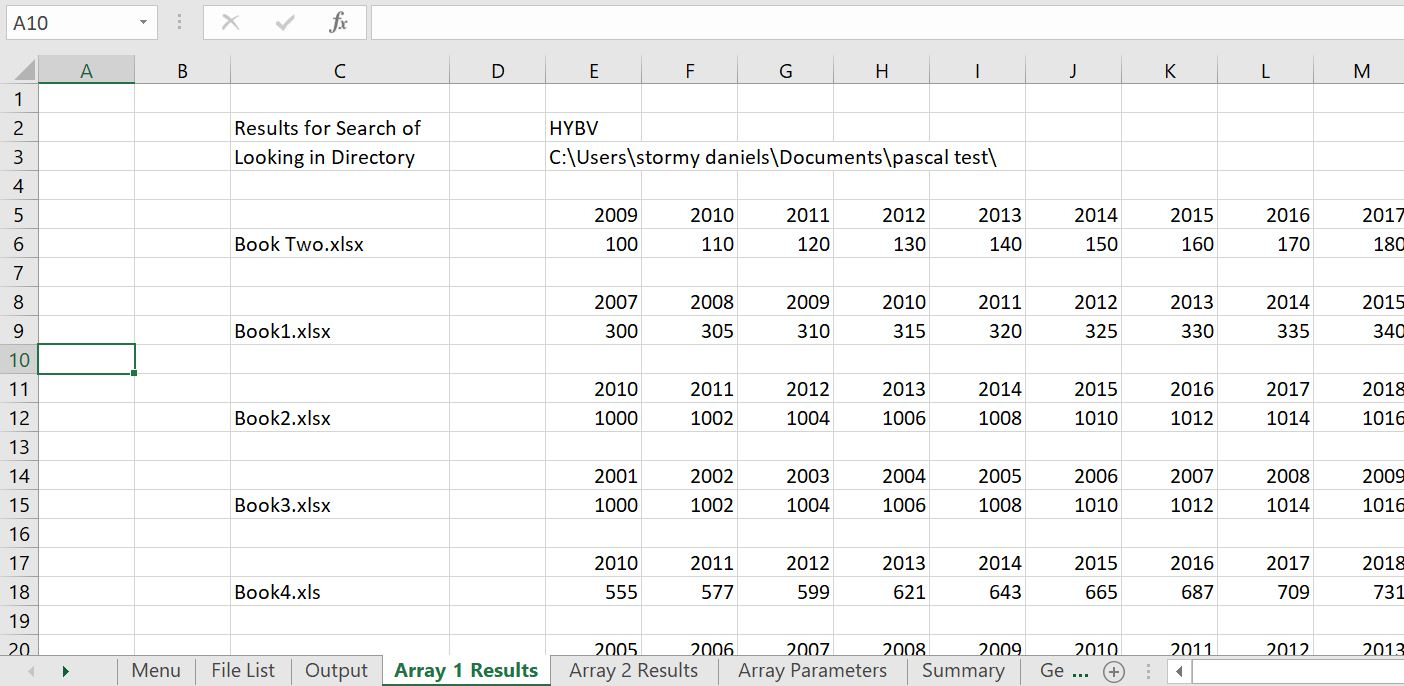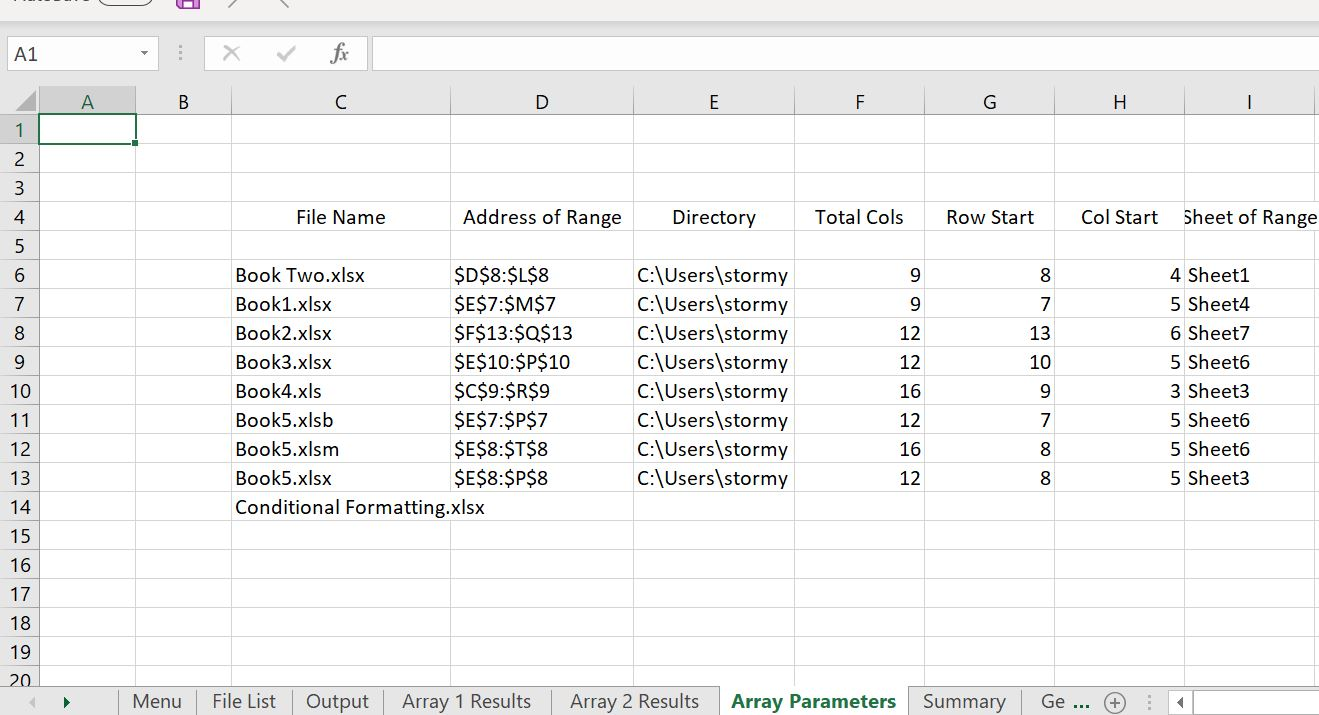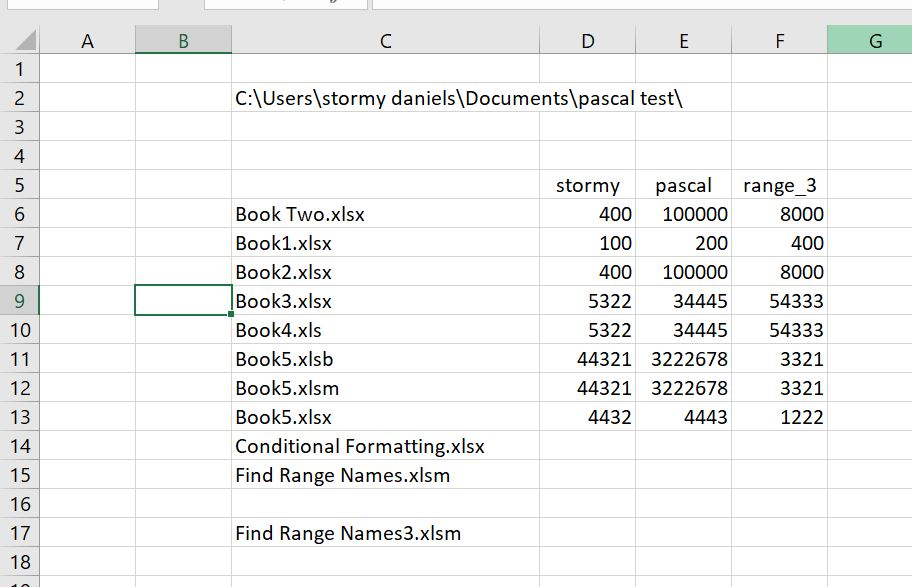.

```Sub LoopThroughFiles()

Dim sFile As String 'File Name
' Dim range1_output As Range

debug_switch = Range("debug_switch")

Application.ScreenUpdating = True ' choose to not show what is going on
If debug_switch = False Then Application.ScreenUpdating = False ' shows what is happening when macro is running

base_book = ActiveWorkbook.Name ' name of this book so you can go back to the file and print etc.
base_sheet = ActiveSheet.Name ' name of this sheet so you can go back to the file and print etc.

Application.ScreenUpdating = True ' choose to not show what is going on

start_row = Range("start_row")
start_col = Range("start_col")

directory_name = Range("directory_name")

If debug_switch = True Then MsgBox " Debug Switch is on"

' loop through each file name and open it if the extension is correct

Count = 0
Count1 = 0

array1 = Range("array_1")
array2 = Range("array_2")
array3 = Range("array_3")

Sheets("Array 1 Results").Select
Range(Cells(Count + start_row, start_col), Cells(Count + start_row + 40, start_col + 40)).ClearContents
Sheets("Array 2 Results").Select
Range(Cells(Count + start_row, start_col), Cells(Count + start_row + 40, start_col + 40)).ClearContents
Sheets("Array Parameters").Select
Range(Cells(Count + start_row, start_col), Cells(Count + start_row + 40, start_col + 40)).ClearContents

sFile = Dir(directory_name)
workbookname = sFile

Sheets("Array 1 Results").Select

Do Until sFile = ""

' Calculate

Workbooks.Open (directory_name & sFile) ' Open the workbook

' On Error Resume Next

Workbooks(base_book).Sheets("Array Parameters").Cells(Count1 + start_row, start_col + 1) = workbookname

On Error GoTo after_while:

Workbooks(base_book).Sheets("Array Parameters").Cells(Count1 + start_row, start_col + 2) = Range(array1).Address
Workbooks(base_book).Sheets("Array Parameters").Cells(Count1 + start_row, start_col + 3) = directory_name
Workbooks(base_book).Sheets("Array Parameters").Cells(Count1 + start_row, start_col + 4) = Range(array1).Columns.Count
Workbooks(base_book).Sheets("Array Parameters").Cells(Count1 + start_row, start_col + 5) = Range(array1).Row
Workbooks(base_book).Sheets("Array Parameters").Cells(Count1 + start_row, start_col + 6) = Range(array1).Column
Workbooks(base_book).Sheets("Array Parameters").Cells(Count1 + start_row, start_col + 7) = Range(array1).Worksheet.Name

cols = Range(array1).Columns.Count ' Number of columns in array so that you can make for loops

' This section is for finding the year

row_cell = Range(array1).Columns.Row
col_cell = Range(array1).Columns.Column

Cells(row_cell, col_cell).Select ' Go to starting cell so you can count upwards
Cells(row_cell, col_cell).Activate

' MsgBox " Beforre Find Year Cell row " & row_cell & " col cell " & col_cell & " value of cell " & Cells(row_cell, col_cell)

For test_row = 1 To 10
test_year = Cells(row_cell - test_row, col_cell)
If test_year > 1980 And test_year < 2020 Then
' MsgBox " Row up Year " & test_year & " test row " & test_row
Exit For
End If
Next test_row

year_row = row_cell - test_row

Workbooks(base_book).Sheets("Array 1 Results").Cells(Count + start_row, start_col + 1) = workbookname
Workbooks(base_book).Sheets("Array 2 Results").Cells(Count + start_row, start_col + 1) = workbookname

For col = 1 To cols
Workbooks(base_book).Sheets("Array 1 Results").Cells(Count + start_row - 1, start_col + 2 + col) = Cells(row_cell - test_row, col_cell + col - 1)
Workbooks(base_book).Sheets("Array 1 Results").Cells(Count + start_row, start_col + 2 + col) = Range(array1).Cells(1, col)
Next col

For col = 1 To cols
Workbooks(base_book).Sheets("Array 2 Results").Cells(Count + start_row - 1, start_col + 2 + col) = Cells(row_cell - test_row, col_cell + col - 1)
Workbooks(base_book).Sheets("Array 2 Results").Cells(Count + start_row, start_col + 2 + col) = Range(array2).Cells(1, col)
Next col

' If Right(sFile, 4) = sExt Then GetInfo sFile

If debug_switch = True Then MsgBox " Closing " & ActiveWorkbook.Name

ActiveWorkbook.Close
' Workbooks(workbookname).Close

Workbooks(base_book).Activate

Resume1:

sFile = Dir ' This is the big one where define the next file name

workbookname = sFile

Count1 = Count1 + 1

Count = Count + 3

Loop

GoTo finish1:

after_while:

MsgBox " No Range Name in File " & worbookname

If debug_switch = True Then MsgBox " Error " & sFile & " " & sFile & " workbook name " & workbookname

' GoTo Resume1

finish1:

Workbooks(base_book).Activate

End Sub```

.